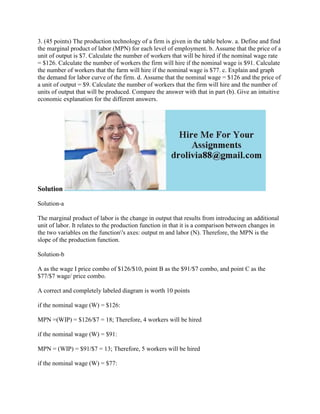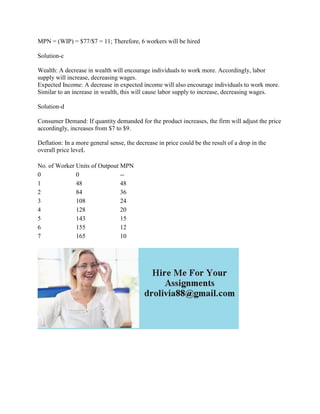Publicité

# 3- (45 points) The production technology of a firm is given in the tab.docx

todd621
8 Feb 2023Prochain SlideShareBreak Even Analysis
Chargement dans ... 3
1 sur 2
Publicité

### 3- (45 points) The production technology of a firm is given in the tab.docx

1. 3. (45 points) The production technology of a firm is given in the table below. a. Define and find the marginal product of labor (MPN) for each level of employment. b. Assume that the price of a unit of output is \$7. Calculate the number of workers that will be hired if the nominal wage rate = \$126. Calculate the number of workers the firm will hire if the nominal wage is \$91. Calculate the number of workers that the farm will hire if the nominal wage is \$77. c. Explain and graph the demand for labor curve of the firm. d. Assume that the nominal wage = \$126 and the price of a unit of output = \$9. Calculate the number of workers that the firm will hire and the number of units of output that will be produced. Compare the answer with that in part (b). Give an intuitive economic explanation for the different answers. Solution Solution-a The marginal product of labor is the change in output that results from introducing an additional unit of labor. It relates to the production function in that it is a comparison between changes in the two variables on the function's axes: output m and labor (N). Therefore, the MPN is the slope of the production function. Solution-b A as the wage I price combo of \$126/\$10, point B as the \$91/\$7 combo, and point C as the \$77/\$7 wage/ price combo. A correct and completely labeled diagram is worth 10 points if the nominal wage (W) = \$126: MPN =(WIP) = \$126/\$7 = 18; Therefore, 4 workers will be hired if the nominal wage (W) = \$91: MPN = (WIP) = \$91/\$7 = 13; Therefore, 5 workers will be hired if the nominal wage (W) = \$77:
2. MPN = (WIP) = \$77/\$7 = 11; Therefore, 6 workers will be hired Solution-c Wealth: A decrease in wealth will encourage individuals to work more. Accordingly, labor supply will increase, decreasing wages. Expected Income: A decrease in expected income will also encourage individuals to work more. Similar to an increase in wealth, this will cause labor supply to increase, decreasing wages. Solution-d Consumer Demand: If quantity demanded for the product increases, the firm will adjust the price accordingly, increases from \$7 to \$9. Deflation: In a more general sense, the decrease in price could be the result of a drop in the overall price leveL No. of Worker Units of Outpout MPN 0 0 -- 1 48 48 2 84 36 3 108 24 4 128 20 5 143 15 6 155 12 7 165 10
Publicité